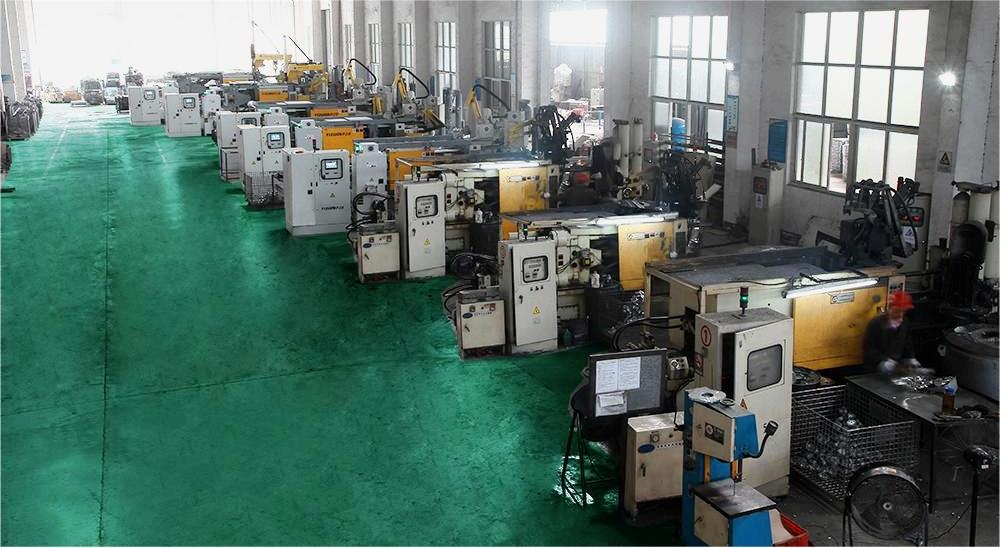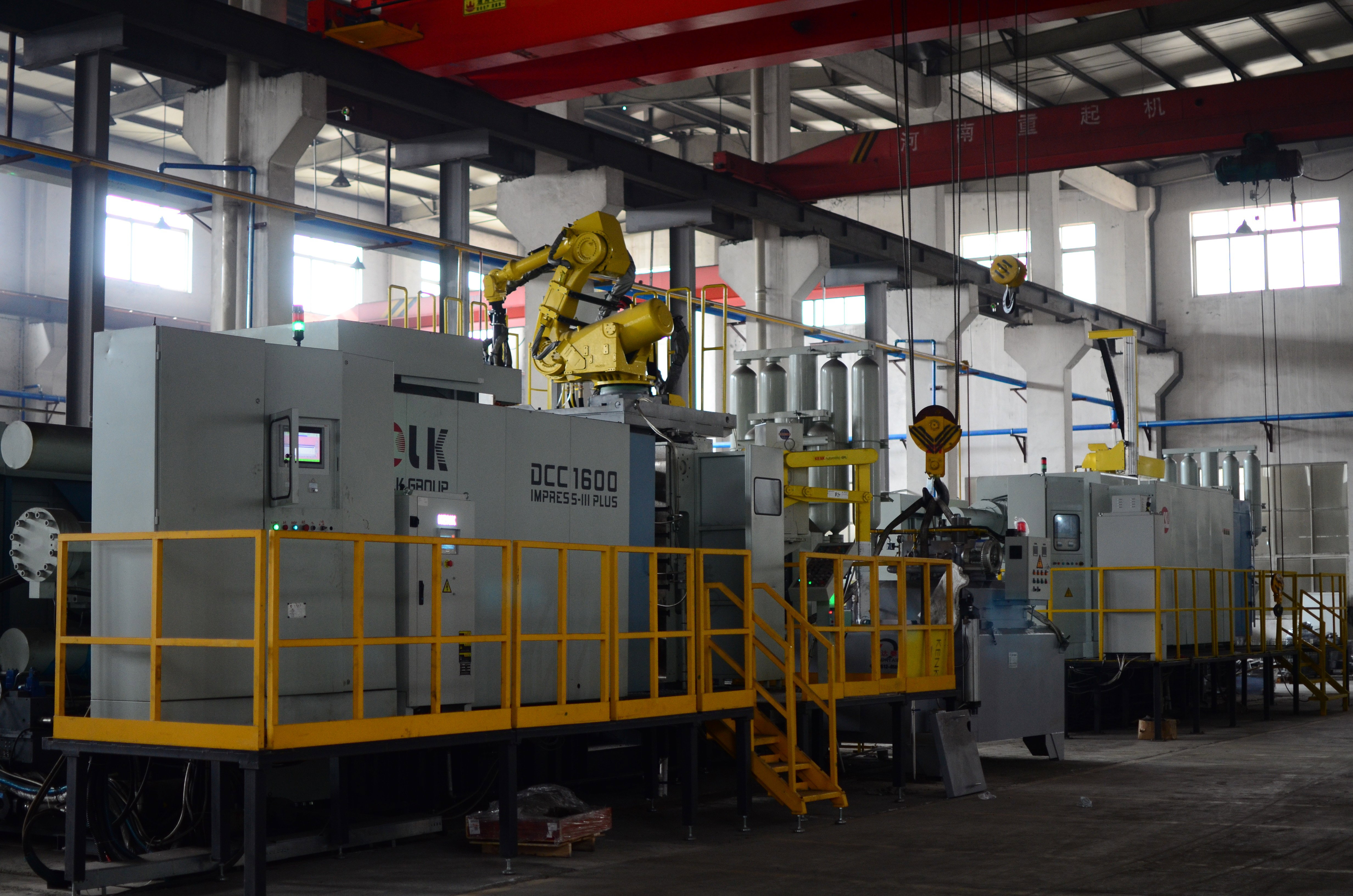21 Jun
21Jun

Some customers may ask us how to choose the tonnage of a die casting machine in high-pressure die casting, and whether higher tonnage is always better. Through this article, we hope to answer customers' questions.

1. Checking Clamping Force

a. Total projection area A = casting projection area A1 + runner area A2 (0.150.3A1) + overflow system area A3 (0.10.2A1) + shot sleeve area A4 (3.14xdxd, where d is the diameter of the shot sleeve, also the same as the punch diameter).

b. Expansion force F1 = A x injection ratio pressure (MPa)
The so-called injection ratio pressure refers to the pressure per unit area, which is supported according to experience: general parts 3050, load-bearing parts 5080, gas-tight parts 80~100.

c. Clamping force F2 = expansion force F1/K, where K is the safety factor, which is usually taken as 0.85. The tonnage of the initial selected die casting machine is determined according to the complexity of the product.

2. Checking Fill Ratio

a. Total weight = casting weight + runner weight + shot sleeve weight + overflow system weight. The projection area of each part is known. After calculating its depth, its volume can be determined.

b. Fill ratio = total weight/casting weight. The casting weight is the maximum alloy weight used when different die casting machines use different shot sleeves. The tonnage of the initial selected die casting machine is checked according to the fill ratio. Usually, the fill ratio is between 40% and 75%.

3. Checking Mold Dimensions

a. Arrange the mold for the casting and determine its mold size.

b. Check whether the selected die casting machine's distance between columns is suitable according to the mold size. Finally, determine the tonnage of the die casting machine to be used.How to choose the tonnage of the die casting machine reasonably:

1. The calculated clamping force must be less than the nominal clamping force of the die casting machine.

2. The calculated chamber volume should be less than the nominal chamber volume of the die casting machine.

3. The calculated mold opening force and ejector force should be less than the maximum mold opening force and ejector force of the selected die casting machine.

4. The total thickness of the mold should be greater than the minimum mold closing distance of the die casting machine.

5. The maximum mold opening distance of the die casting should be greater than the sum of the total thickness of the mold and the height of the product.

6. The size and hole position of the mold plate installed on the die casting machine should be compatible with the size and hole position of the fixed plate of the die casting machine.

7. The maximum size of the outer shape of the mold and the external structure of the extended mold body should not collide with the tie rods of the die casting machine, otherwise, it will affect the normal operation and use of the die casting machine.

8.Determine the clamping force of the die casting machine

--Calculate clamping force
--Safety factor
--Injection ratio pressure
--Projection area

The safety factor K is related to the complexity of the casting and the die casting process. Generally, it is taken as 1-1.3. For thin-walled and complex castings, due to the use of higher injection speed, injection ratio pressure, and mold temperature, the parting surface of the mold is subjected to greater impact, so K should be taken as a larger value, and vice versa.2. Determine the shot volume of the machine

The shot volume is an important parameter of the die casting machine device. It is the most commonly used parameter when selecting a die casting machine and is expressed in kilograms. Choose a die casting machine with sufficient shot volume - should not choose a die casting machine with a shot volume that is just equal to the weight of the casting blank. Usually, the weight of the casting blank should account for 25% to 85% of the shot volume.

3. Determine the projection area of the part

The projection area is an important parameter for selecting the die casting machine for the casting. The projection area here is not only the area of the part but also includes the area of the waste gate and the sprue. The area is estimated by dividing the clamping force by the injection ratio pressure during die casting. For the calculation of the casting area and casting surface area, it should be divided into small regular shapes.
a. Calculation of the surface area of a circle with a diameter of d: s=πd2/4.
b. Calculation of the surface area of a square with a side length of a: s=a2.
c. Calculation of the surface area of a rectangle with a length of a and a width of b: s=ab.
d. Calculation of the surface area of a trapezoid with an upper base of a, a lower base of b, and a height of h: s=(a+b)h/2.

Conclusion: Choosing the appropriate tonnage of a die casting machine is crucial in high-pressure die casting and should be comprehensively considered based on factors such as the projection area, expansion force, fill ratio, and mold dimensions. Selecting a die casting machine with a tonnage that is too small can result in unstable casting quality, while choosing a machine with a tonnage that is too large can waste resources and increase production costs. Therefore, for different products, the appropriate tonnage of the die casting machine should be selected reasonably based on factors such as complexity and size.